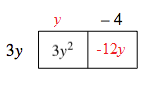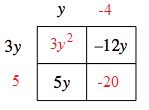### Home > GB8I > Chapter 4 Unit 5 > Lesson INT1: 4.2.2 > Problem4-76

4-76.

For each of the following area models, determine the dimensions (length and width) and write the area as a product and as a sum. Homework Help ✎

1.  $-4$ $3y$ $3y^2$
• Find the length and width of each small rectangle.

•• $3y(y−4)=3y^2−12y$

1.  $y$ $3y$ $-12y$ $5y$

• Follow the steps in part (a).

•• $(3y+5)(y−4)=3y^2−7y−20$